# midas Structure

BLOG Tips & Tutorials

# Response Spectrum Analysis

The dynamic equilibrium equation for a structure subjected to a ground motion used in a response spectrum analysis can be expressed as follows: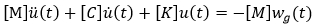Where,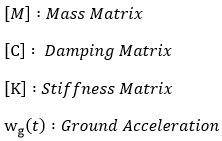and,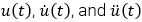are relative displacement, velocity, and acceleration respectively.

Response spectrum analysis assumes the response of a multi-degree-of-freedom (MDOF) system as a combination of multiple single-degree-of-freedom (SDOF) systems. A response spectrum defines the peak values of responses corresponding to and varying with natural periods (or frequencies) of vibration that have been prepared through a numerical integration process. Displacements, velocities, and accelerations form the basis of a spectrum. Response spectrum analyses are generally carried out for seismic designs using the design spectra defined in design standards.

To predict the peak design response values, the maximum response for each mode is obtained first and then combined by an appropriate method. For seismic analysis, the displacement and inertial force corresponding to a particular degree of freedom for the m-th mode are expressed as follows:

<EQ. 1>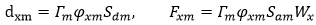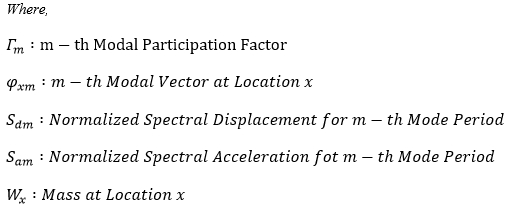In a given mode, the spectral value corresponding to the calculated natural period is searched from the spectral data through linear interpolation. It is therefore recommended that spectral data at closer increments of natural periods be provided at the locations of curvature changes (refer to Figure 1). The range of natural periods for spectral data must be sufficiently extended to include the maximum and minimum natural periods obtained from the eigenvalue analysis.

Some building codes indirectly specify the seismic design spectral data by means of dynamic coefficient, foundation factor, zoning factor, importance factor, etc. MIDAS/Gen can generate the design spectrum using these seismic parameters. However, the ductility factor (or Response modification factor or Seismic response factor) is typically applied at the member design stage.

Response spectrum analyses are allowed in any direction on the Global X-Y plane and in the vertical Global Z direction. You may choose an appropriate method of modal combination for analysis results such as the Complete Quadratic Combination (CQC) method or the Square Root of the Sum of the Squares (SRSS) method.

The following describes the methods of modal combination:

SRSS (Square Root of the Sum of the Squares)

<EQ. 2>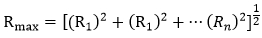ABS (Absolute Sum)

<EQ. 3>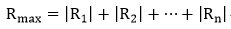<EQ. 4>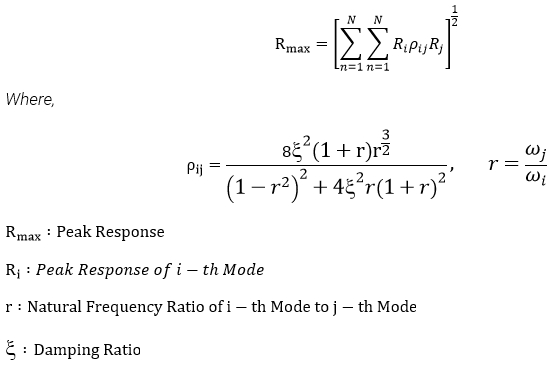In ,<Eq. 4>, when i = j, then ρij =1 regardless of the damping ratio. If the damping ratio (ξ) becomes zero (0), both CQC and SRSS methods produce identical results.

The ABS method produces the largest combination values among the three methods. The SRSS method has been widely used in the past, but it tends to overestimate or underestimate the combination results in the cases where the values of natural frequencies are close to one another. As a result, the use of the CQC method is increasing recently as it accounts for probabilistic interrelations between the modes.

If we now compare the displacements of each mode for a structure having 3 DOF with a damping ratio of 0.05, the results from the applications of SRSS and CQC are as follows:

Natural Frequencies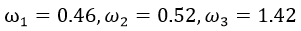Maximum Modal Displacements: Dij (displacement components of i-th degree of freedom for j-th mode)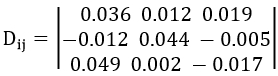If SRSS is applied to compute the modal combination for each degree of freedom,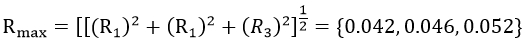If CQC is applied,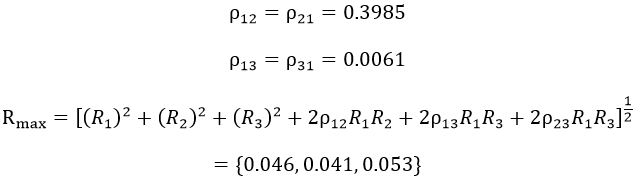Comparing the two sets of displacements for each degree of freedom, we note that the SRSS method underestimates the magnitude for the first degree of freedom overestimates the value for the second degree of freedom relative to those obtained by CQC. Thus, the SRSS method should be used with care when natural frequencies are close to one another.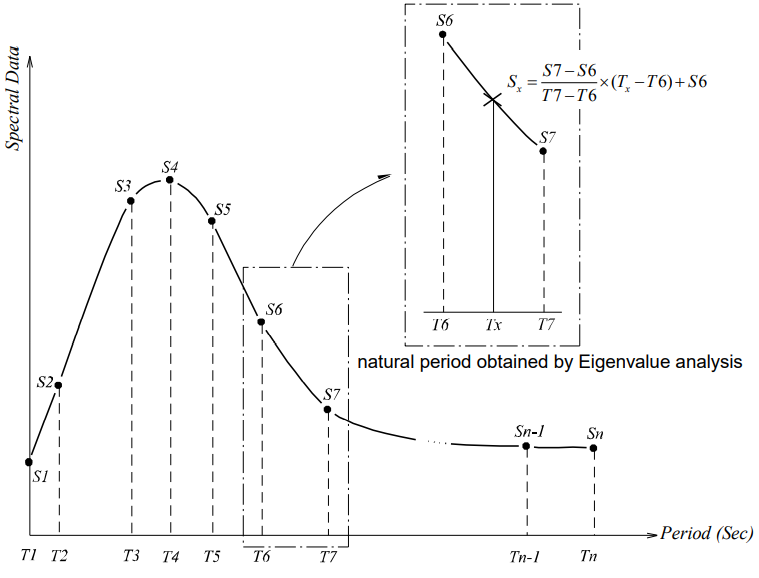Figure 1. Response Spectrum Curve and Linear Interpolation of Spectral Data

# Time-History Analysis

Mode shapes and natural periods of an undamped free vibration are obtained from the characteristic equation below.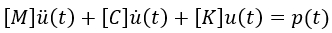Where,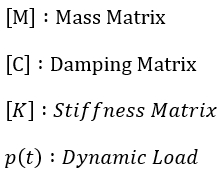and,are displacement, velocity, and acceleration respectively.

Time history analysis seeks out a solution for the dynamic equilibrium equation when a structure is subjected to dynamic loads. It calculates a series of structural responses (displacements, member forces, etc.) within a given period of time-based on the dynamic characteristics of the structure under the applied loads. MIDAS/Gen uses the Modal Superposition Method for time history analysis.

## Modal Superposition Method

The displacement of a structure is obtained from a linear superposition of modal displacements, which maintain orthogonal characteristics to one another. This method premises on the basis of that the damping matrix is composed of a linear combination of the mass and stiffness matrices as presented below.

<EQ. 1>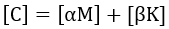<EQ. 2>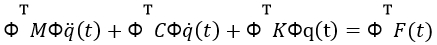<EQ. 3>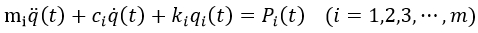<EQ. 4>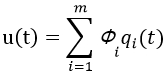<EQ. 5>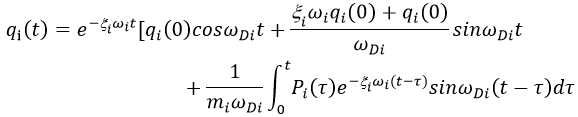Where,
<EQ. 6>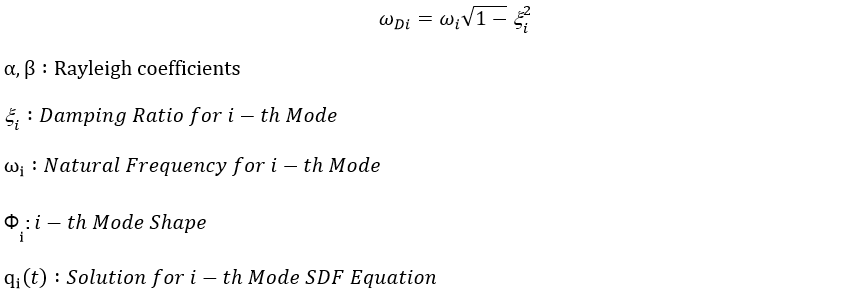When a time history analysis is carried out, the displacement of a structure is determined by summing up the product of each mode shape and the solution for the corresponding modal equation as expressed in <Eq. 4>. Its accuracy depends on the number of modes used.

This modal superposition method is very effective and, as a result, widely used in linear dynamic analyses for large structures. However, this method cannot be applied to nonlinear dynamic analyses or to the cases where damping devices are included such that the damping matrix cannot be assumed as a linear combination of the mass and stiffness matrices.

The following outlines some precautions for data entries when using the modal superposition method:

Total Analysis Time (or Iteration Number)

Time Step

Time step can affect the accuracy of analysis results significantly. The increment must be closely related to the periods of higher modes of the structure and the period of the applied force. The time step directly influences the integral in <Eq. 5>, and as such specifying an improper time step may lead to inaccurate results. In general, one-tenth of the highest modal period under consideration is reasonable for the time step. In addition, the time step should be smaller than that of the applied load.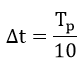where, Tp=the highest modal period being considered.

Modal Damping Ratios (or Rayleigh Coefficients)

Values for determining the energy dissipation (damping) properties of a structure, which relate to either the total structure or individual modes.

Dynamic loads are directly applied to nodes or foundations of a structure, which are expressed as a function of time. The change of loadings must be well represented in the forcing function. A loading at an unspecified time is linearly interpolated.

Figure 2 shows an idealized system to illustrate the motion of a SDOF structural system. The equilibrium equation of motion subjected to forces exerting on a SDOF system is as follows:

<EQ. 7>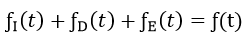fI(t) is an inertia force, which represents a resistance to the change of velocity of a structure. The inertia force acts in the opposite direction to the acceleration, and its magnitude is mü(t). ƒE(t)  is an elastic force by which the structure restores its configuration to the original state when the structure undergoes a deformation. This force acts in the opposite direction to the displacement, and its magnitude is ku(t). ƒD(t). is a damping force, which is a fictitious internal force dissipating kinetic energy and thereby decreasing the amplitude of a motion. The damping force may come in a form of internal frictions. It acts in the opposite direction to the velocity, and its magnitude is cü(t).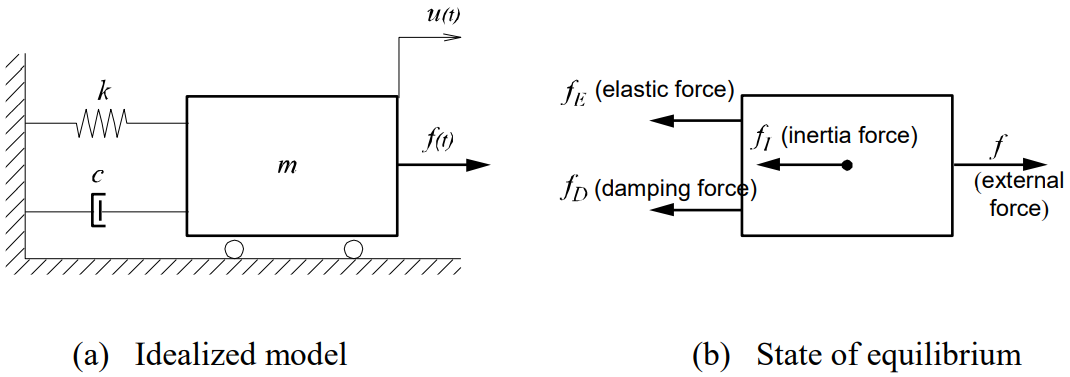Figure 2. The Motion of SDOF System

The above forces are now summarized as

<EQ. 8>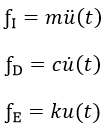where, m, c, and k represent mass, damping coefficient and elastic coefficient respectively. From the force equilibrium shown in Figure 2(b), we can obtain the equation of motion for a SDOF structural system.

<EQ. 9><Eq. 9> becomes the equation of damped free vibration by letting f(t)=0, and it becomes the equation of undamped free vibration if the condition of c=0 is additionally imposed on the damped free vibration. If f(t) is assigned as a seismic loading (or displacements, velocities, accelerations, etc.) with varying time, the equation then represents a forced vibration analysis problem. The solution can be found by using either Modal Superposition Method or Direction Integration Method.

# Buckling Analysis

Linear buckling analysis is used to determine Critical Load Factors and the corresponding Buckling Mode Shapes of a structure, which is composed of truss, beam or plate elements. The static equilibrium equation of a structure at a deformed state is expressed as

<EQ. 1>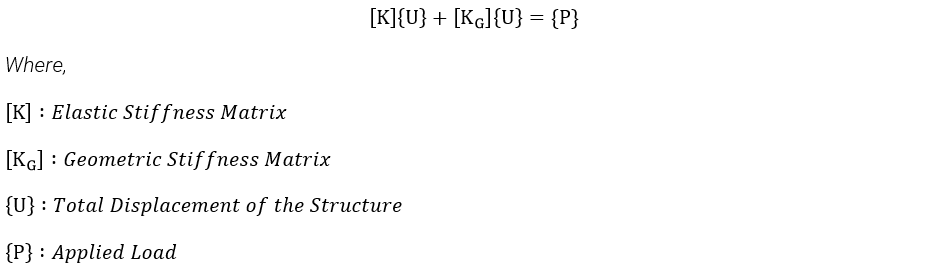The geometric stiffness matrix of a structure can be obtained by summing up the geometric stiffness matrix of each element. The geometric stiffness matrix in this case represents a change in stiffness at a deformed state and is directly related to the applied loads. For instance, a compressive force on a member tends to reduce the stiffness, and conversely, a tensile force tends to increase the stiffness.

* If you would like to keep reading the Buckling Analysis, fill out the form below and download WHITE PAPER for MIDAS/Gen Analysis Options - Part 2.😉

Author InformationYoung-Wook Hur | Technical Manager, MIDAS IT

Young-Wook has over 8+ years of experience in building design, especially apartment, complex and palnt structures. He is currently working at MIDAS IT as a technical manager, mainly assisting with various projects related to structural engineering.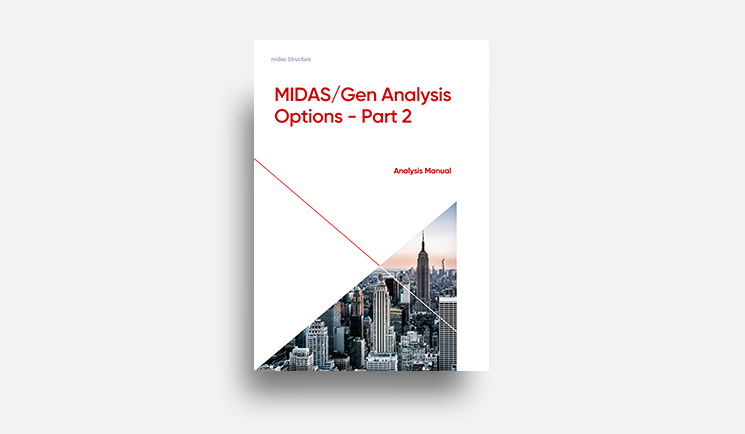E-BOOK MIDAS/Gen Analysis
Options - Part 2.

In this material, you can deeply learn how to perform 3 representative analyses:
1. Response Spectrum Analysis
2. Time-History Analysis
3. Buckling Analysis